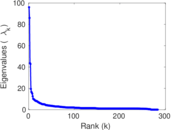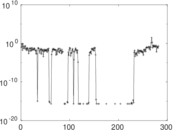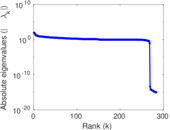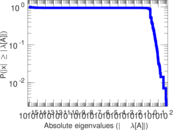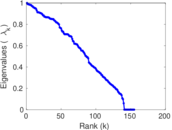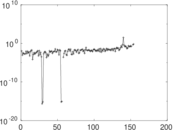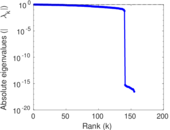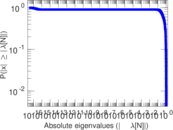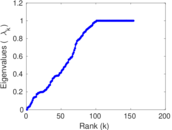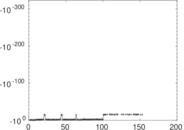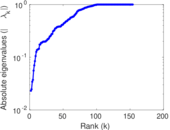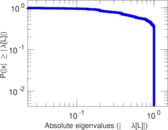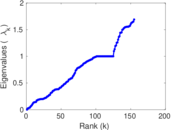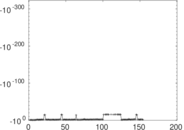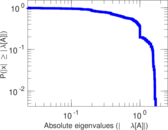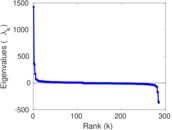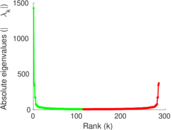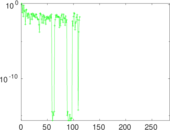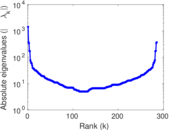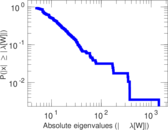# Wikiquote edits (af)

This is the bipartite edit network of the Afrikaans Wikiquote. It contains users and pages from the Afrikaans Wikiquote, connected by edit events. Each edge represents an edit. The dataset includes the timestamp of each edit.

 Code `qaf` Internal name `edit-afwikiquote` Name Wikiquote edits (af) Data source http://dumps.wikimedia.org/ AvailabilityDataset is available for download Consistency checkDataset passed all tests Category Authorship network Dataset timestamp 2017-10-20 Node meaning User, article Edge meaning Edit Network formatBipartite, undirected Edge typeUnweighted, multiple edges Temporal dataEdges are annotated with timestamps

## Statistics

 Size n = 1,438 Left size n1 = 285 Right size n2 = 1,153 Volume m = 3,450 Unique edge count m̿ = 2,133 Wedge count s = 172,580 Claw count z = 22,948,473 Cross count x = 2,725,601,556 Square count q = 43,506 4-Tour count T4 = 1,044,926 Maximum degree dmax = 942 Maximum left degree d1max = 942 Maximum right degree d2max = 149 Average degree d = 4.798 33 Average left degree d1 = 12.105 3 Average right degree d2 = 2.992 19 Fill p = 0.006 491 08 Average edge multiplicity m̃ = 1.617 44 Size of LCC N = 1,119 Diameter δ = 14 50-Percentile effective diameter δ0.5 = 3.596 50 90-Percentile effective diameter δ0.9 = 6.149 71 Median distance δM = 4 Mean distance δm = 4.336 31 Gini coefficient G = 0.733 423 Balanced inequality ratio P = 0.200 435 Left balanced inequality ratio P1 = 0.144 638 Right balanced inequality ratio P2 = 0.270 145 Relative edge distribution entropy Her = 0.817 148 Power law exponent γ = 3.463 88 Tail power law exponent γt = 2.231 00 Tail power law exponent with p γ3 = 2.231 00 p-value p = 0.000 00 Left tail power law exponent with p γ3,1 = 1.831 00 Left p-value p1 = 0.167 000 Right tail power law exponent with p γ3,2 = 2.421 00 Right p-value p2 = 0.000 00 Degree assortativity ρ = −0.259 279 Degree assortativity p-value pρ = 4.149 52 × 10−34 Spectral norm α = 95.996 6 Algebraic connectivity a = 0.023 076 9 Spectral separation |λ1[A] / λ2[A]| = 1.116 64 Controllability C = 882 Relative controllability Cr = 0.622 003

## Plots

### Fruchterman–Reingold graph drawing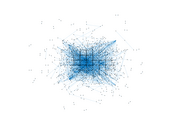### Degree distribution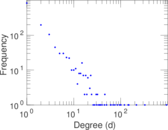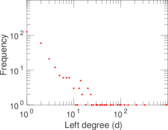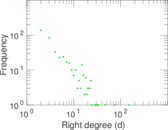### Cumulative degree distribution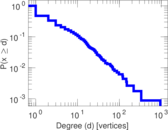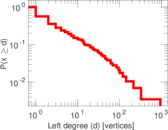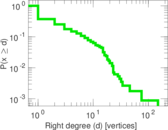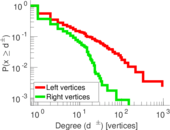### Lorenz curve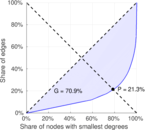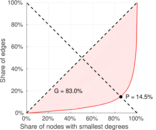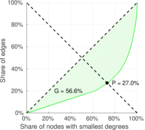### Spectral distribution of the adjacency matrix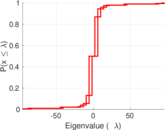### Spectral distribution of the normalized adjacency matrix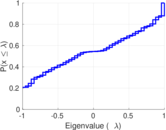### Spectral distribution of the Laplacian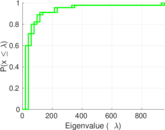### Spectral graph drawing based on the adjacency matrix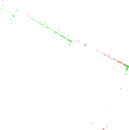### Spectral graph drawing based on the Laplacian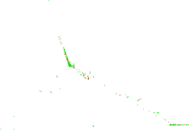### Spectral graph drawing based on the normalized adjacency matrix### Degree assortativity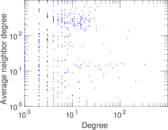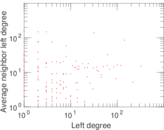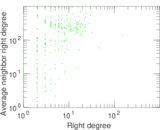### Zipf plot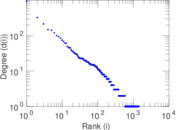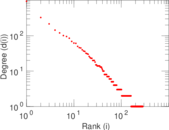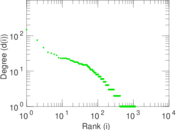### Hop distribution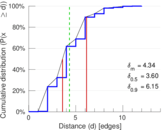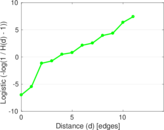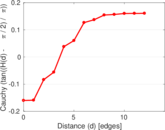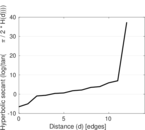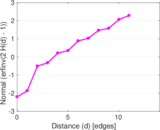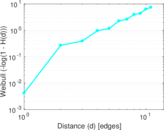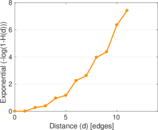### Double Laplacian graph drawing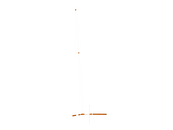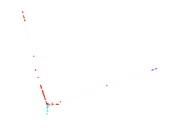### Delaunay graph drawing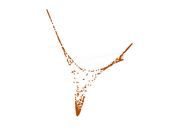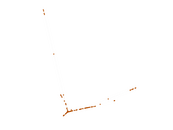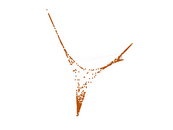### Edge weight/multiplicity distribution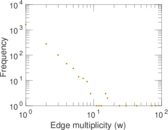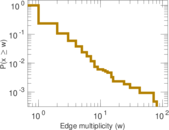### Temporal distribution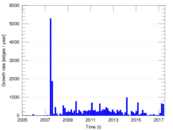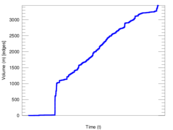### Temporal hop distribution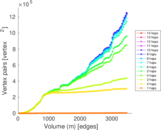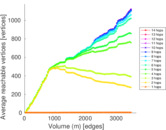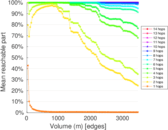### Diameter/density evolution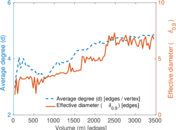### Matrix decompositions plots